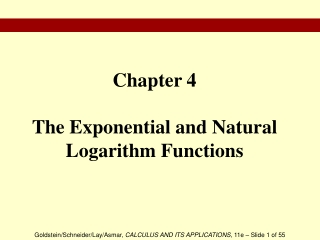DownloadDownload PresentationChapter 4 The Exponential and Natural Logarithm Functions

# Chapter 4 The Exponential and Natural Logarithm Functions

Download Presentation## Chapter 4 The Exponential and Natural Logarithm Functions

- - - - - - - - - - - - - - - - - - - - - - - - - - - E N D - - - - - - - - - - - - - - - - - - - - - - - - - - -
##### Presentation Transcript

1. Chapter 4The Exponential and Natural Logarithm Functions

2. Chapter Outline • Exponential Functions • The Exponential Function ex • Differentiation of Exponential Functions • The Natural Logarithm Function • The Derivative ln x • Properties of the Natural Logarithm Function

3. §4.1 Exponential Functions

4. Section Outline • Exponential Functions • Properties of Exponential Functions • Simplifying Exponential Expressions • Graphs of Exponential Functions • Solving Exponential Equations

5. Exponential Function

6. Properties of Exponential Functions

7. Simplifying Exponential Expressions EXAMPLE Write each function in the form 2kx or 3kx, for a suitable constant k. SOLUTION (a) We notice that 81 is divisible by 3. And through investigation we recognize that 81 = 34. Therefore, we get (b) We first simplify the denominator and then combine the numerator via the base of the exponents, 2. Therefore, we get

8. Graphs of Exponential Functions Notice that, no matter what b is (except 1), the graph of y = bx has a y-intercept of 1. Also, if 0 < b < 1, the function is decreasing. If b > 1, then the function is increasing.

9. Solving Exponential Equations EXAMPLE Solve the following equation for x. SOLUTION This is the given equation. Factor. Simplify. Since 5x and 6 – 3x are being multiplied, set each factor equal to zero. 5x≠ 0.